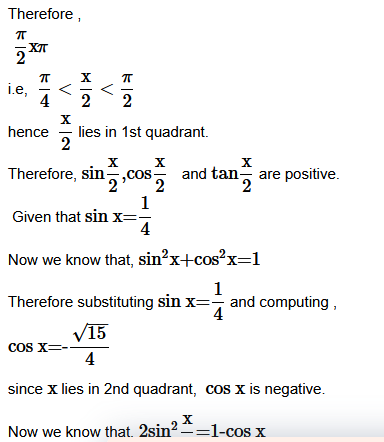# CLASS 11 MATHS CHAPTER-3 TRIGONOMETRIC FUNCTIONS

### Exercise 3.1

Question1. Find the radian measures corresponding to the following degree measures:

(i)25

(ii)−47 30′

(iii)240

(iv)520

Solution :

(i)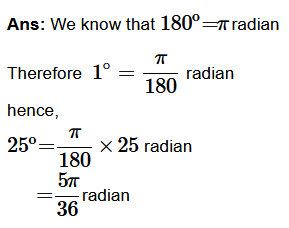(ii)

(iii)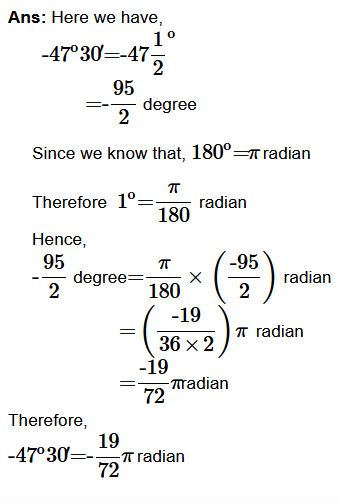(iv)

Question2. Find the degree measures corresponding to the following radian measures (Use π=22/7).

(i)11/16

(ii)−4

(iii)5π/3

(iv)7π/6

Solution :

(I)

(ii)

Question3. A wheel makes 360 revolutions in one minute. Through how many radians does it turn in one second?

Solution :
Number of revolutions in 1 minute = 360

Number of revolutions the wheel makes in 1 minute=360

Number of revolutions the wheel make in 1
second=360/60
=6

In one complete revolution, the wheel turns an angle of 2 π

Hence, it will turn an angle of 6 × 2 π =12 π

complete revolutions.

Therefore, the wheel turns an angle of 12 π

Question4. Find the degree measure of the angle subtended at the centre of a circle of radius 100 cm by an arc of length 22 cm (Use π=22/7).

Solution :

We know that,
in a circle of radius r unit, if  an angle  θ  radian at the centre is subtended by an arc of length l unit then.

Question5. In a circle of diameter 40 cm, the length of a chord is 20 cm. Find the length of minor arc of the chord.

Solution :

Therefore, the length of the minor arc of the chord is 20 π/ 3cm .

Question6. In the two circles , arcs of the same length subtend angles 60ºand75º at the centre, find the ratio of their radii.

Solution :Ans: Let the radii of the two circles be r1 and r2 . Let an arc of length l1 subtends an angle of 60º at the centre of the circle of radius r1 , whereas let an arc of length l2 subtends an angle of 75o at the centre of the circle of radius r2

.

Now, we have,

60º= π / 3 radian  and

75º=5π/12

We know that,

in a circle of radius r
unit, if  an angle  θ  radian at the centre is subtended by an arc of length l

unit then

θ = l/r

l = r θ

Hence we obtain,

l = rπ/ 3

and  l = r25 π / 12

according to the l1=l2

thus we have,

r1 π / 3 π =r25 π 12

r1=r25 / 4

r1 / r2 =5 / 4

Hence , the ratio of the radii is 5:4

Question7. Find the angle in radians through which a pendulum swings if its length is 75 cm and the tip describes an arc of length:

(i) 10 cm

(ii) 15 cm

(iii) 21 cm

Solution :
(i) We know that,

in a circle of radius r
unit, if  an angle  θ  radian at the centre is subtended by an arc of length l

unit then

θ = l / r

Given that r=75cm

And here, l=10cm

Hence substituting the values in the formula,

θ =10 / 75

=215
.

(ii) Given: length of pendulum r = 75 cm and length of arc l = 15 cm

θ =15 / 75

θ =1 / 5

(iii) Given: length of pendulum r = 75 cm and length of arc l = 21 cm

θ =21 / 75

θ =7 / 25

### Exercise 3.2

Question1. Find the values of other five trigonometric functions in cosx = −1/2 , x lies in third quadrant.

Solution :
Given:

cosx = −1/2 ,

sec x = 1/cosx

Substituting the values

=  1/ (-1/2) = -2

Question2. Find the values of other five trigonometric functions in sinx = 3/5  , x lies in second quadrant.

Solution :
Given:

sinx = 3/5

Question3. Find the values of other five trigonometric functions in cotx = 3/4  , x lies in third quadrant.

Solution :
Given:

cotx = 3/4

Question4. Find the values of other five trigonometric functions in  secx= 13/5  , lies in fourth quadrant.

Solution :
Given: secx= 13/5  ,

We can write  it as

cos x = 1 / secx = 1/ (13/5)

= 5 / 13

we know that

sin2 x + cos2 x = 1

sin2 x  = 1 – cos2 x

putting the value

sin2 x = 1 – (5/13)2

sin2 x = ± 12 /13 [4th lies in fourth quadrant]

Now  sinx = -12 /13

we can write as

cosec x = 1 /sinx = 1 /(-12/13) = -13/12

so we get

tanx = sin x / cos x =(-12/13)/(5/13) = -12/5

Question5. Find the values of other five trigonometric functions in  tanx= −5/12 , x lies in second quadrant..

Solution :
Given: Here given that,  tan x = -5 / 12

Therefore we have,
cot x = 1/tan x

=1/(-5/12)

=-12/5

Now we know that , sec2x – tan2x =1

Therefore we have, sec2x = 1+ tan2x

Substituting  tan x=-5/12

in the formula, we obtain,

sec2x =1+(-5/12)2

sec2x=1+25 / 144

=169 / 144

sec x= ± 13/12

Since x
lies in the 2nd quadrant, the value of secx

will be negative.

sec x=-13/12

Therefore, cos x = 1/sec x

= -12/13

Now  , tan x = sin x/cos x

Therefore, sin x = tan x/cos x

Hence we have, sin x=(-5/12) × (-12/13)

=(5/13)

And

cosec x=1/sin x

=135

Find the values of the trigonometric functions in exercises 6 o 10.

Question6. sin 765

Solution :
Here We know that the values of sinx repeat after an interval of 2π or 360º

Therefore we can write,

sin765º=sin(2 × 360º+45º)

=sin45º

=√1/2.

Question7. cosec (−1410).

Solution :
Here We  know that the values of cosec x repeat after an interval of 2 π  or 360º
Therefore we can write,

cosec(-1410º)=cosec(-1410º+4 × 360º)

=cosec(-1410º+1440º)

=cosec30º

=2

Question8. tan 19π/3.

Solution :
Here We know that the values of tan x repeat after an interval of  π  or 180º
Therefore we can write,

tan 19π/ 3=tan6×1/3 π

=tan(6 π + π/ 3)

=tan π/ 3

=√3

9. sin (−11π/3)

Solution :
Here We know that the values of sin x repeat after an interval of 2 π  or 360º

Therefore we can write,

sin(-11π / 3)=sin(-11π / 3 + 2 × 2 π )

=sin( π /3)

=√3/2

Question10. cot (−15π/4).

Solution :
Here We  know that the values of cot x repeat after an interval of  π  or 180∘
Therefore we can write,

cot(-15 π / 4)=cot(-15 π /4+4 π )

=cot π /4

=1

### Prove that:

Question1. Prove that: sin2 π/6 + cosπ/3 − tanπ/4 = −1/2

Question2. Prove that: 2sin2 π/6  +  cosec7π/6 cos2 π/3 =3/2

Question3. Prove that: cot2 π/6  +  cosec 5π/6  +  3tan2 π/6 = 6

Solution :
L.H.S. = Substituting the values of  cot π / 6, cosec5 π / 6, tan π / 6
on left hand side,

L.H.S .= cot2 π/ 6 + cosec 5π / 6 + 3tan 2π/ 6

=(√3)2+cosec( π – π /6) + 3(1/√3)2
=3+cosec π /6 + 3 × 1/3

Since cosec x repeat its value after an interval of 2 π ,

we have, cosec5 π/ 6  = cosec π / 6
L.H.S =3+2+1=1
=R.H.S.

Hence proved.

Question4. Prove that: 2sin2 3π/4 + 2cos2 π/4 + 2sec2 π/3 =10

Solution :

Question5. Find the value of:

(i) sin75
(ii) tan15

Solution :
(i) sin75 = sin(45∘ + 30) = sin45 cos30 + cos45 sin30

Since we know that, sin(x+y)=sin x cos y+cos x sin y
Therefore we have,

Sin75º=1 / √2 × √3/2 + 1/√2– 1 / 2

Sin75º=(√3 + 1) /2√2

(ii)

Prove the following:

Question6.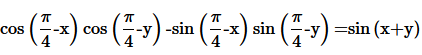Question7.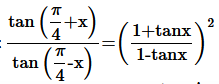Solution :

Question8.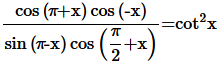Solution :

Question9.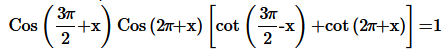Solution :

Question10. Prove the following: sin(n+1)x  sin(n+2)x  +  cos(n+1)x cos(n+2)x = cosx

Solution :We know that , cos(x-y)=cosxcosy+sinxsiny
L.H.S.=sin(n+1)xsin(n+2)x+cos (n+1)x cos (n+2)x

=cos[(n+1)x-(n+2)x]
=cos(-x)
=cosx
=R.H.S.

Question11.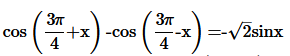Question12. Prove the following: sin2 6x − sin2 4x = sin 2x sin 10x

Ans.

Question13. Prove the following: cos2 2x − cos2 6x = sin 4x sin 8x

Solution :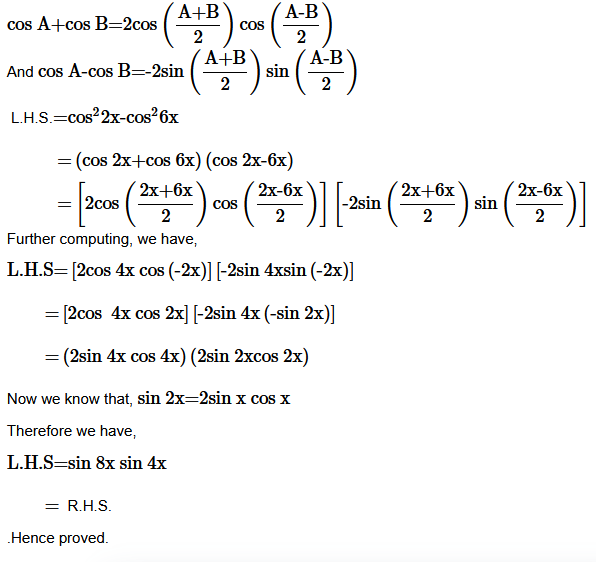Question14. Prove the following: sin2x + 2sin4x + sin6x = 4cos2x sin4x

Question15. Prove the following: cot 4x (sin 5x + sin 3x) = cot x (sin 5x – sin 3x)

Solution :

Question16. Prove the following:

Solution :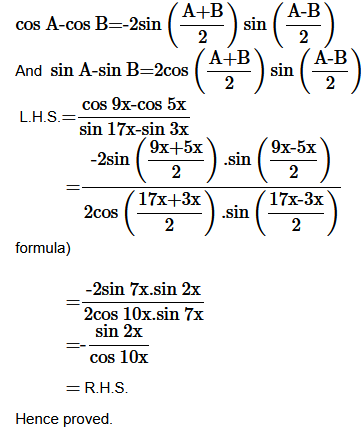Question17.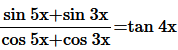Solution :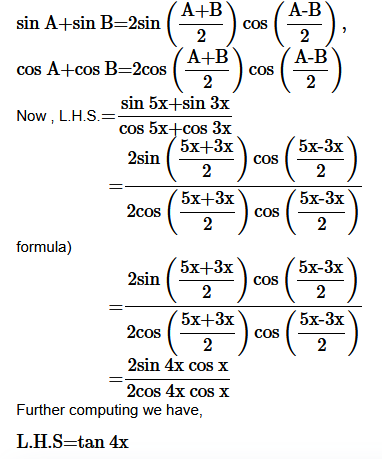.

Question18.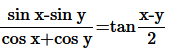Solution :

.

Question19.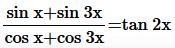Solution :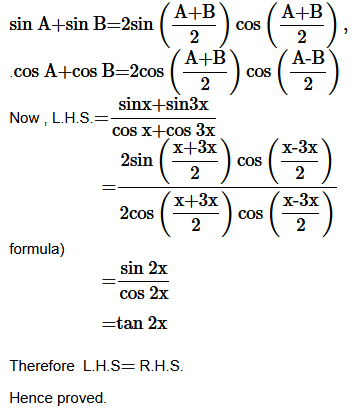.

Question20.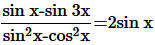Solution :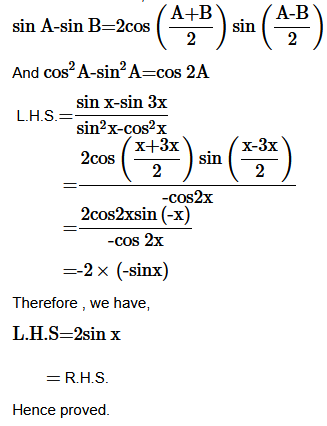Question21.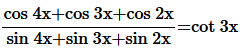Solution :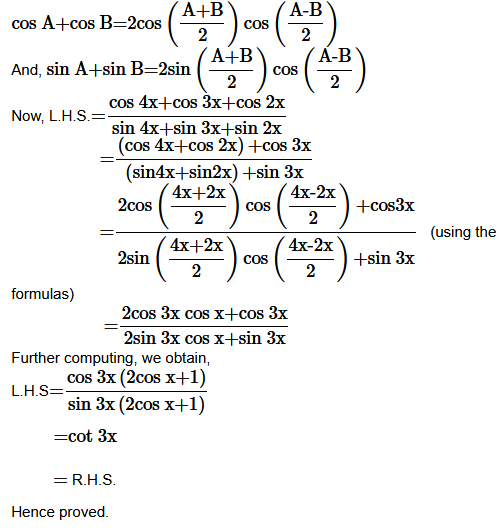Question22. Prove the following: cot x cot 2x − cot 2x cot 3x − cot 3x cot x =1

Solution :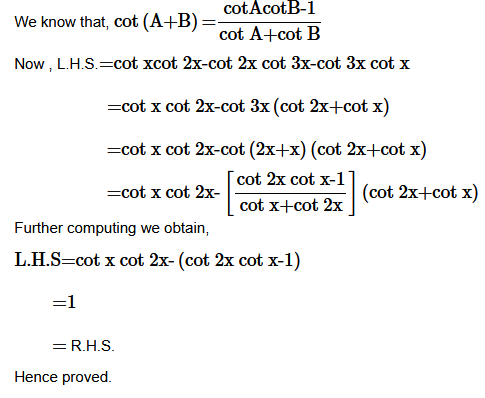Question23.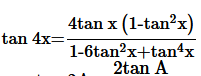Question24. Prove the following: cos 4x = 1 − 8sin2 x cos2 x

Ans. L.H.S. = cos 4x

= cos 2 (2x)

= 1 – 2 sin22x

= 1 – 2(2sin x cos x)2

computing we get,

L H S  = 1 – 8sin2xcos2x

= RHS

Hence proved

Question25. Prove the following: cos6 x = 32cos6 x − 48cosx + 18cos2 x−1

Ans. L.H.S. = cos 6x

= cos 3 (2x)

= 4cos32x – 3cos 2x

= 4[ (2cos2x – 1 )3 – 3(2cos2x-1)]

= L.H.S=4[(2cos2x)3-(1)3-3(2cos2x)]-6cos2x + 3

=4[(2cos2x)3-(1)3-3(2cos2x)2+3(2cos2x)]-6cos2x+3

=4[8cos6x-1-12cos4x+6cos2x]-6cos2x+3

=32cos6x-48cos4x+18cos2x-1= R.H.S.

### Exercise 3.4

Find the principal and general solutions of the following equations:

Question1. tan x = √3

Solution :
Given: tan x = √3Here lies in first or third quadrant.

tan x = √3
We know that  tan π/ 3 = √3
and tan(4 π/ 3)=tan( π + π 3)=tan π / 3 = √3
Therefore, the principal solutions are x = π /3
and 4π / 3
Now, tan x = tan π/ 3
Which implies,
x=nπ + π / 3
where n∈Z
Therefore, the general solution is x=n π + π /3
, where n∈Z .

Question2. Sec x = 2

Solution :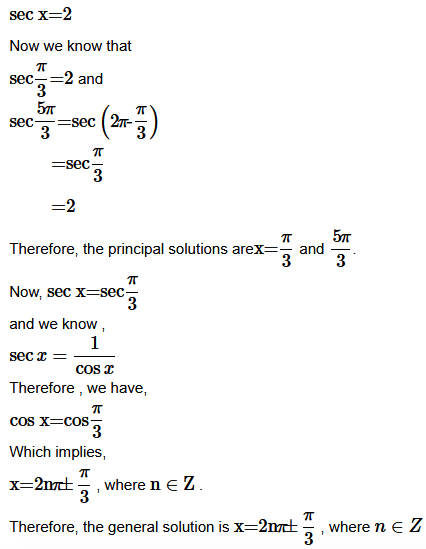Question3. Cot x = −√3

Solution :

cot x = -√3
Now we know that  cot π / 6 = √3

And cot( π – π /6) = -cot π/ 6

=-3–√
and  cot(2 π – π 6)=-cot π 6

=-√3
Therefore we have,

cot5π / 6 = -√3
and cot 11π/ 6=-√3

Therefore, the principal solutions are x=5 π/ 6
and 11 π /6
Now, cot x = cot5 π/ 6
And we know cot x=1 / tan x

Therefore we have,

tan x = tan5 π / 6

Which implies,

x = n π + 5 π /6
, where n∈Z

Therefore, the general solution is x=nπ + 5 π /6
, where n∈Z .

Question4. Cosec x = -2

Solution :
Given: Cosec x = -2

cosec π/6 = 2

and

Therefore, the general solution is x=n π +(-1)7π / 6  ,where n∈Z.

Question5. Find the general solution for each of the following equations: cos 4 x = cos 2 x

Solution :
Given: cos 4 x = cos 2 x

Question6. Find the general solution for each of the following equations: cos 3x + cos x – cos 2x = 0

Solution :
Given: cos 3x + cos x – cos 2x = 0

Question7. Find the general solution for each of the following equations: sin 2x + cos x = 0

Solution :
Given: sin 2x + cos x = 0

Now we know that, sin 2x = 2sin x cos x
Therefore we have,
2sinx  cos x + cos x=0
Which implies,

cos x(2sin x+1)=0

Therefore we have,

Either cos x=0
or sin x = -1/2

Hence  we have ,

x = (2n + 1) π /2 , where n ∈ Z

Question8. Find the general solution for each of the following equations: sec2 2x = 1−tan 2x

Solution :
Given: sec2 2x = 1−tan 2x

Now we know that

sec2 2x – tan 2x = 1

Ther for we have ,

sec2 2x = 1− tan 2x

Question9. Find the general solution for each of the following equations: sin x + sin 3x + sin 5x = 0

Solution :
Given: sin x + sin 3x + sin 5x = 0

### Miscellaneous Exercise

Prove that:

Question1. Prove that: 2cos π/13 cos 9π/13 + cos 3π/13 + cos 5π/13 = 0

Solution :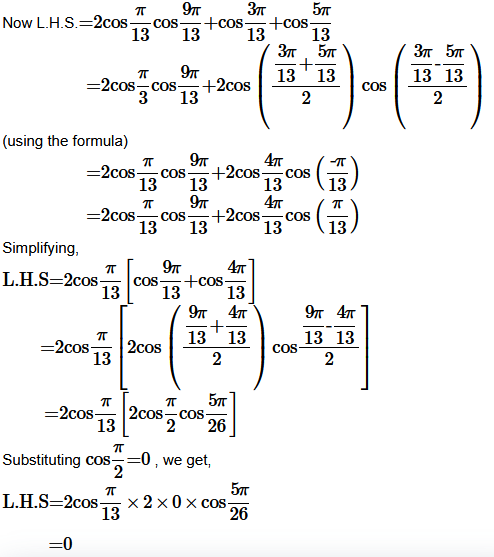0 = R.H.S.

Question2. Prove that: (sin 3x + sin x) sin x + (cos 3x – cos x) cos x = 0

Solution :
L.H.S. = (sin 3x + sin x) sin x + (cos 3x – cos x) cos x

Question3. Prove that: (cosx + cosy)+ (sinx − siny)2 = 4cos2 x /2 + y/2

Solution :
L.H.S. = (cosx + cosy)+ (sinx − siny)2

Question4. (cosx − cosy)2 + (sinx − siny)2 = 4sin2 x/2 − y/2

Solution :
L.H.S. = (cosx − cosy)2 + (sinx − siny)2

Question5. Prove that: sin x + sin 3x + sin 5x + sin 7x = 4 cos x cos 2x sin 4x

Solution :
L.H.S. = sinx + sin3x + sin5x + sin7x = (sinx + sin5x) + (sin3x + sin7x)

Question6.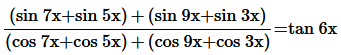Solution :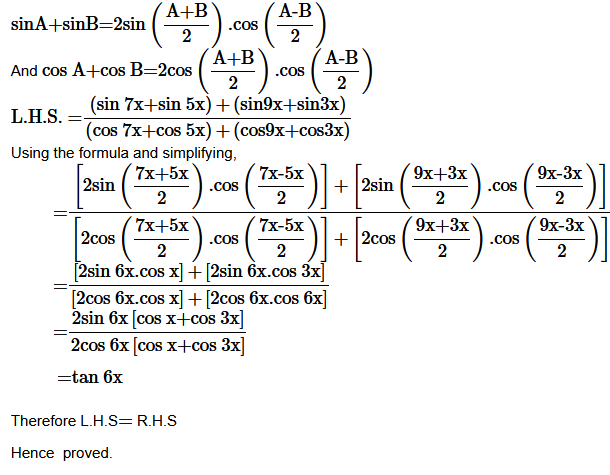Question7. Prove that: sin 3x + sin 2x − sin x = 4sin x cos x/2 cos3 x/2

Solution :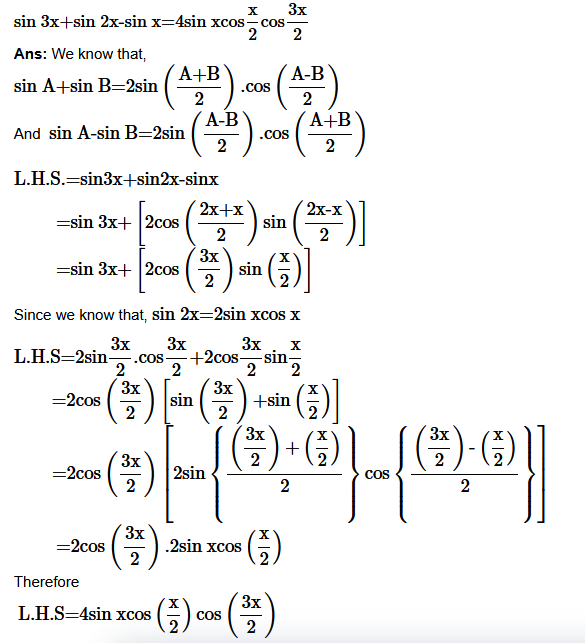Question8 Find sin x/2,cos x/2 and tan x/2 in each of the following:

Solution :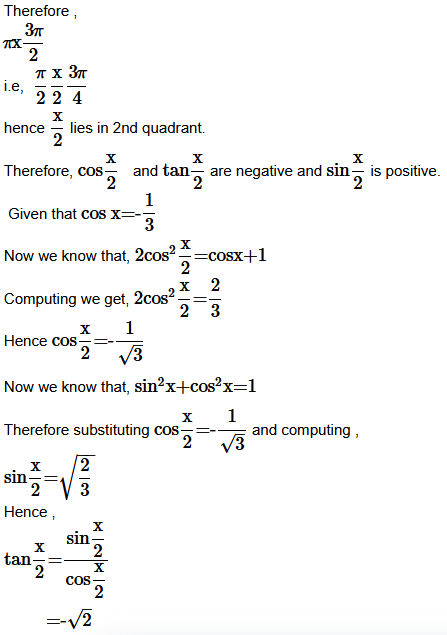Solution :